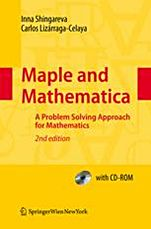### Logowanie

Zapomniałeś hasła?
Nowy klient

Jeżeli nie znalazłeś poszukiwanej książki, skontaktuj się z nami wypełniając formularz kontaktowy.

Ta strona używa plików cookies, by ułatwić korzystanie z serwisu. Mogą Państwo określić warunki przechowywania lub dostępu do plików cookies w swojej przeglądarce zgodnie z polityką prywatności.

### Informacje szczegółowe o książce# Maple and Mathematica, 2nd ed. 2009

##### ISBN 9783211994313

Autor: Inna K. Shingareva

Wydawca: Springer

Dostępność: Wysyłka w ciągu 2-3 dni

Cena: 313,95 zł

 ISBN13: 9783211994313 ISBN10: 3211994319 Autor: Inna K. Shingareva Oprawa: Paperback Rok Wydania: 2009-09-01 Numer Wydania: 2 Ilość stron: 484

The first book to compare the main two computer algebra systems (CAS), Maple and Mathematica used by students, mathematicians, scientists, and engineers. Both systems are presented in parallel so that Mathematica users can learn Maple quickly by finding the Maple equivalent to Mathematica functions, and vice versa. This student reference handbook consists of core material for incorporating Maple and Mathematica as a working tool into different undergraduate mathematical courses (abstract and linear algebra, geometry, calculus and analysis, complex functions, special functions, integral and discrete transforms, algebraic and transcendental equations, ordinary and partial differential equations, integral equations, numerical analysis and scientific computing). The book also contains applications from various areas of mathematics, physics, and music theory and can be useful for graduate students, professors, and researchers in science and engineering.
One of the goals of this book is to develop problem-solving skills (that are most useful for solving sophisticated research problems) finding solutions with Maple and Mathematica and not to depend on a specific version of both systems (Maple 12 and Mathematica 6 and 7 are considered).

Part I, describes the foundations of Maple and Mathematica (with equivalent problems and solutions). Part II, describes Mathematics with Maple and Mathematica by using equivalent problems.
Finally, this book is ideal for scientists who want to corroborate their Maple and Mathematica work with independent verification provided by another CAS.
J. Carter, SIAM Review 50: 149-152 (2008).

Preface.- Foundations of Maple and Mathematica: Maple; Mathematica.- Mathematics: Maple and Mathematica: Graphics; Algebra; Linear Algebra; Geometry; Calculus and Analysis; Complex Functions; Special Functions and Orthogonal Polynomials; Integral and Discrete Transforms; Mathematical Equations; Numerical Analysis and Scientific Computing.-

References.- General Index.- Maple Index.- Mathematica Index.- CD-ROM Contents.

### Koszyk

Książek w koszyku: 0 szt.

Wartość zakupów: 0,00 zł

### Kontakt

Gambit
Centrum Oprogramowania
i Szkoleń Sp. z o.o.

Al. Pokoju 29b/22-24

31-564 Kraków

+48 12 414 3791

+48 12 414 3387

Siedziba Księgarni

ul. Kordylewskiego 1

31-542 Kraków

+48 12 410 5989

+48 12 414 3767

Wyślij e-mail

### Subskrypcje

Administratorem danych osobowych jest firma Gambit COiS Sp. z o.o. Na podany adres będzie wysyłany wyłącznie biuletyn informacyjny.

### Autoryzacja płatności